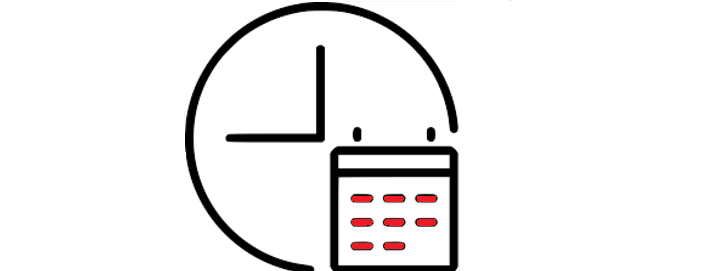Python Tutorial

# Python Timestamp Examples

A Python timestamp is a representation of a point in time as the number of seconds that have elapsed since January 1, 1970, at 00:00:00 UTC. This date and time is known as the "epoch", and serves as a reference point for all timestamps. In Python, timestamps are typically represented as float values, with precision to the microsecond.

## Get the curent timestamp in Python

You can obtain the current timestamp in Python using the time module's time() function.

import time timestamp = time.time() print(timestamp) //Output: 1676015691.8487642

Above code will output the current timestamp as a float value. To format the timestamp as a human-readable string, you can use the datetime module

## Convert timestamp to a datetime

You can convert a timestamp to a datetime object in Python using the datetime module's datetime class and the fromtimestamp method. The fromtimestamp method takes a timestamp as input and returns a corresponding datetime object.

import time import datetime timestamp = time.time() dt_object = datetime.datetime.fromtimestamp(timestamp) print(dt_object) //Output: 2023-02-10 07:55:25.943600

This will output the current date and time as a datetime object, which can be formatted as a string using the strftime method.

import time import datetime timestamp = time.time() dt_object = datetime.datetime.fromtimestamp(timestamp) print(dt_object.strftime("%Y-%m-%d %H:%M:%S")) //Output: 2023-02-10 07:55:52

Above code will output the current date and time as a string in the format YYYY-MM-DD HH:MM:SS. The strftime method takes a format string as input and returns a formatted string representation of the datetime object. You can customize the format string to get the desired output.

## Convert datetime to timestamp

You can convert a datetime object to a timestamp in Python using the timestamp method of the datetime class. The timestamp method returns the timestamp as a float representing the number of seconds since the epoch (January 1, 1970, at 00:00:00 UTC).

import datetime dt_object = datetime.datetime.now() timestamp = dt_object.timestamp() print(timestamp) //Output: 1676015772.763685

Above code will output the current date and time as a timestamp in seconds as a floating-point number. Note that the datetime.now() method is used to get the current date and time as a datetime object, but you can also use any other datetime object as input.

## Convert timestamp to a datetime using calendar Module

The calendar module in Python also provides functionality to work with timestamps. One way to use the calendar module to convert a timestamp to a datetime object is by using the gmtime function. This function takes a timestamp as input and returns a corresponding time tuple, which can be passed to the datetime class constructor to create a datetime object.

import time import calendar import datetime timestamp = time.time() time_tuple = time.gmtime(timestamp) dt_object = datetime.datetime(*time_tuple[:6]) print(dt_object) //Output: 2023-02-10 07:56:35

Above code will output the current date and time as a datetime object, which can be formatted as a string using the strftime method, as shown in the previous section.

## Convert Timestamp to StringConvert a timestamp to a string in Python, you first need to convert the timestamp to a datetime object, and then use the strftime method to format the datetime object as a string.

import time import datetime timestamp = time.time() dt_object = datetime.datetime.fromtimestamp(timestamp) date_string = dt_object.strftime("%Y-%m-%d %H:%M:%S") print(date_string) //Output: 2023-02-10 07:57:25

Above code will output the current date and time as a string in the format YYYY-MM-DD HH:MM:SS. The strftime method takes a format string as input and returns a formatted string representation of the datetime object. You can customize the format string to get the desired output.

## Get Timestamp in Milliseconds

In order to get the timestamp in milliseconds in Python, you need to multiply the value returned by the time.time() function by 1000. This will give you the timestamp in seconds multiplied by 1000, which represents the number of milliseconds since the epoch (January 1, 1970, at 00:00:00 UTC).

import time timestamp_milliseconds = int(round(time.time() * 1000)) print(timestamp_milliseconds) //Output: 1676015863606

Above code will output the current timestamp in milliseconds as an integer value. Note that the value is rounded to the nearest integer using the round function, and then converted to an integer using the int function.

## Get the Current Date and Time using timestamp

To get the current date and time in Python using a timestamp, you can use the time module to get the current time as a timestamp, and then use the datetime module to convert the timestamp to a datetime object.

import time import datetime timestamp = time.time() dt_object = datetime.datetime.fromtimestamp(timestamp) print(dt_object) //Output: 2023-02-10 07:58:05.569645

Above code will output the current date and time as a datetime object. You can then use the strftime method to format the datetime object as a string, as I mentioned in my previous answers.

### Conclusion

A timestamp represents a point in time, usually measured in seconds since the Unix Epoch (January 1, 1970, at 00:00:00 UTC). Python provides various ways to work with timestamps, such as using the time module to access time-related functions or the datetime module for more advanced date and time operations, making it easy to manage and manipulate time-related data in Python applications.